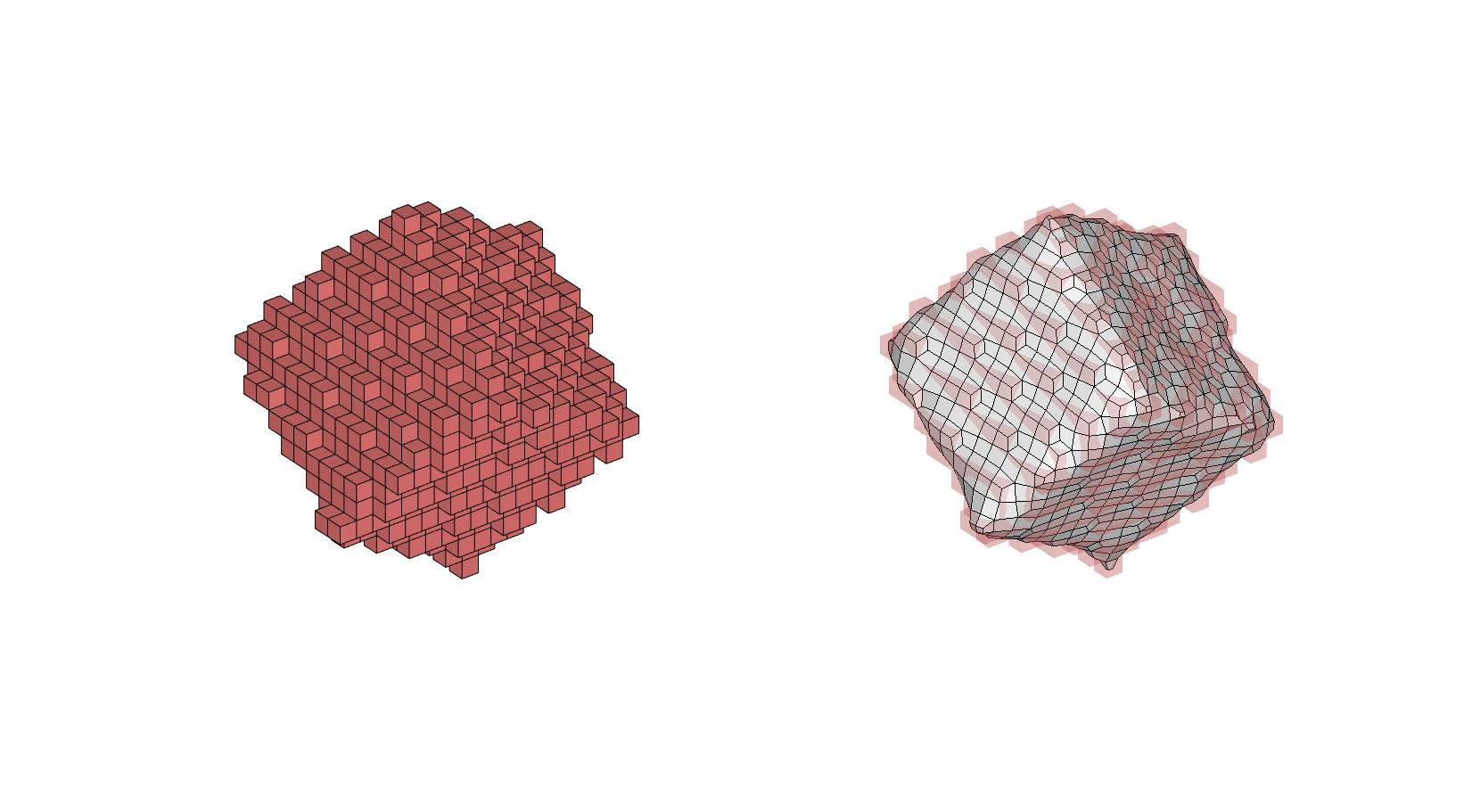# smoothTaubin

Below is a demonstration of the features of the smoothTaubin function

## Contents

```clear; close all; clc;
```

## Syntax

[Vs]=smoothTaubin(F_vox,V_vox,nSmooth,lambda,mu);

## Description

Function and documentation are a work in progress, consider helping.

## Examples

```testCase=1;
switch testCase
case 1
boxDim=[10 10 10];
pointSpacing=2;
[F_ori,V_ori]=triBox(boxDim,pointSpacing);
R=euler2DCM(pi/4*ones(1,3));
V_ori=V_ori*R;
case 2
[F_ori,V_ori]=graphicsModels(5);
end
```
```pointSpacing=mean(patchEdgeLengths(F_ori,V_ori));

% Using |patch2Im| function to convert patch data to image data
[M,G,~]=patch2Im(F_ori,V_ori,[],pointSpacing/2.5);

[F_vox,V_vox,~]=im2patch(M,M==1,'vb');

voxelSize=G.voxelSize;
imOrigin=G.origin;
[V_vox(:,1),V_vox(:,2),V_vox(:,3)]=im2cart(V_vox(:,2),V_vox(:,1),V_vox(:,3),voxelSize*ones(1,3));

V_vox=V_vox+imOrigin(ones(size(V_vox,1),1),:);
```
```lambda=0.5
k_pb=0.1
mu = 1./(k_pb-(1/lambda))
k=2;
f=(1-k.*lambda).*(1-mu.*k);

nSmooth=50;

[Vs]=smoothTaubin(F_vox,V_vox,nSmooth,lambda,mu);
```
```lambda =

0.5000

k_pb =

0.1000

mu =

-0.5263

```
```cFigure;
subplot(1,2,1);
% gpatch(F_ori,V_ori,'kw','none',0.5);
gpatch(F_vox,V_vox,'rw','k',1);

subplot(1,2,2);
gpatch(F_vox,V_vox,'rw','none',0.25);
gpatch(F_vox,Vs,'w','k',1);

gdrawnow;
```GIBBON www.gibboncode.org

Kevin Mattheus Moerman, [email protected]

GIBBON footer text

GIBBON: The Geometry and Image-based Bioengineering add-On. A toolbox for image segmentation, image-based modeling, meshing, and finite element analysis.

Copyright (C) 2006-2022 Kevin Mattheus Moerman and the GIBBON contributors

This program is free software: you can redistribute it and/or modify it under the terms of the GNU General Public License as published by the Free Software Foundation, either version 3 of the License, or (at your option) any later version.

This program is distributed in the hope that it will be useful, but WITHOUT ANY WARRANTY; without even the implied warranty of MERCHANTABILITY or FITNESS FOR A PARTICULAR PURPOSE. See the GNU General Public License for more details.

You should have received a copy of the GNU General Public License along with this program. If not, see http://www.gnu.org/licenses/.